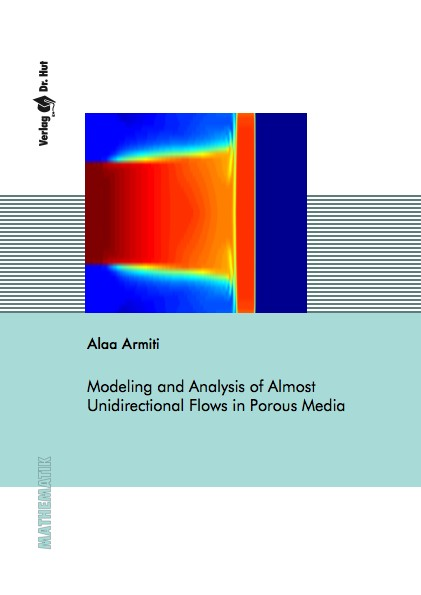Datenbestand vom 20. August 2019aktualisiert am 20. August 2019

# ISBN 9783843934336Euro 72,00 inkl. 7% MwSt

978-3-8439-3433-6, Reihe Mathematik

Alaa Armiti
Modeling and Analysis of Almost Unidirectional Flows in Porous Media

157 Seiten, Dissertation Universität Stuttgart (2017), Softcover, A5

## Zusammenfassung / Abstract

For many environmental and industrial applications, such as aquifers purification and extraction of fossil fuels from reservoirs, it is crucial to study fluid flows in porous media and the phenomenon of saturation overshoots. Typically, mathematical models are used to describe and predict such flows. These models are often coupled systems of nonlinear differential equations. As a result, exact solutions are hard to obtain and only numerical simulations are feasible. In this thesis, we contribute to three aspects of modeling transport processes in the subsurface: first, reducing models' complexity based on natural properties, second, describing more physical phenomena like saturation overshoots, and third, proving models' well-posedness.

In saturated media, fluid flows are modeled using the coupled two-phase flow model. Applying this model in large media might lead to very high computational complexity. However, transport processes in saturated media are in general almost horizontal, such as the flow of extracted oil. This natural property has been utilized to reduce model's complexity. Of specific interest is the approach proposed by Yortsos (1995), which reduces the number of unknowns. In this thesis, we perform several numerical examples to analyze the validity and numerical efficiency of this approach. We also extend it to describe almost horizontal flows in media with high porosity as well as macroscopically heterogeneous media, where fluids velocity is often modeled using Brinkman's equations instead of Darcy's law. Our extended approach leads to a third-order pseudo-parabolic differential equation of saturation only. We provide various numerical tests to show the computational efficiency of the extended model. Additionally, these tests show the ability of the model to describe the phenomenon of saturation overshoots. Finally, we investigate the well-posedness of the extended model, where we prove the existence of weak solutions and the uniqueness for a special case of it.

In unsaturated soils, the vertical infiltration processes are modeled using Richards' equation, which is a second-order parabolic equation of saturation. This equation is unable to describe saturation overshoots, as it satisfies the maximum principle. Therefore, different higher-order extensions have been suggested. We propose a nonlinear fourth-order extension of Richards' equation and prove its well-posedness.